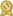Found 3800 calculus tutors – page 5 of 380.

Birmingham 35224 · \$35/hour · teaches Algebra 2 - Calculus - SAT Math

Dear Parents and Students, I am privileged to assist you all. I have excelled in math throughout my life. I am well versed in arithmetic ranging from basic math all the way up to calculus I. I can help out with calculus...certified

Houston 77095 · \$35/hour · teaches Algebra 1 - Calculus - Elementary (K-6th) - MATLAB -

My areas of expertise include pre-algebra, algebra, pre-calculus, and calculus 1. I have also taken calculus 2, calculus 3, and differential equations, so I have a strong aptitude for math.certified

Richmond 23222 · \$35/hour · teaches Algebra 2 - Calculus - Differential Equations -

...management (Business pre calculus, and calculus) and Math (Algebra, pre calculus, Calculus I, II & III, Mathemcertified

Bryan 77808 · \$50/hour · teaches Business - Calculus - Differential Equations -

While in college, I took many math based courses such as engineering physics, calculus, calculus II, calculus III, differential equations, mathematical economics, and more. I started tutoring Spanish, calculus...certified

Mound 55364 · \$40/hour · teaches Algebra 2 - Calculus - Geometry - Prealgebra -

I developed the AP calculus program in my school. I have taught AP Calculus AB for 21 years and BC calculus for 13 years. I have been an AP Reader for both AB and BC calculus for the past 5 years.certified

Jacksonville 32246 · \$30/hour · teaches C - Calculus - Computer Programming - Computer

I can help you with Pre-Calculus, Calculus 1, Calculus 2, Calculus 3, Linear Algebra, Probability & Statistics, Modeling and Simulation, and Numerical Methods. I also can be of assistance in preparing you...certified

Pomona 91767 · \$49/hour · teaches C++ - Calculus - Elementary Math - Elementary Science

I have successfully prepared students for SAT and ACT Math tests, and up to Trigonometry/Pre-calculus classes. In high school, I was in an accelerated math program where I was able to take AP Calculus...certified

Westlake 44145 · \$30/hour · teaches Algebra 2 - Calculus - Chemistry - Organic

The best way to teach someone is to make him think. I have been tutoring for the past two years. I tutored geometry, algebra, and calculus for most my students, and I also have experience tutoring physics and chemistry.certified

Sugar Land 77498 · \$50/hour · teaches Algebra 2 - Calculus - Common Core - Elementary

My area of specialization includes electrical engineering, electronics, maths, pre-algebra, pre calculus, calculus 1, Calculus II and Calculus III, science, algebra, and trigonometry.certified

San Jose 95123 · \$50/hour · teaches C++ - Calculus - Chemistry - Computer Engineering -

Math: I have several years experience tutoring/teaching Algebra 2 , Pre-calculus, Calculus, and Linear Algebra. In college, I have taught algebra, pre-calculuscertified